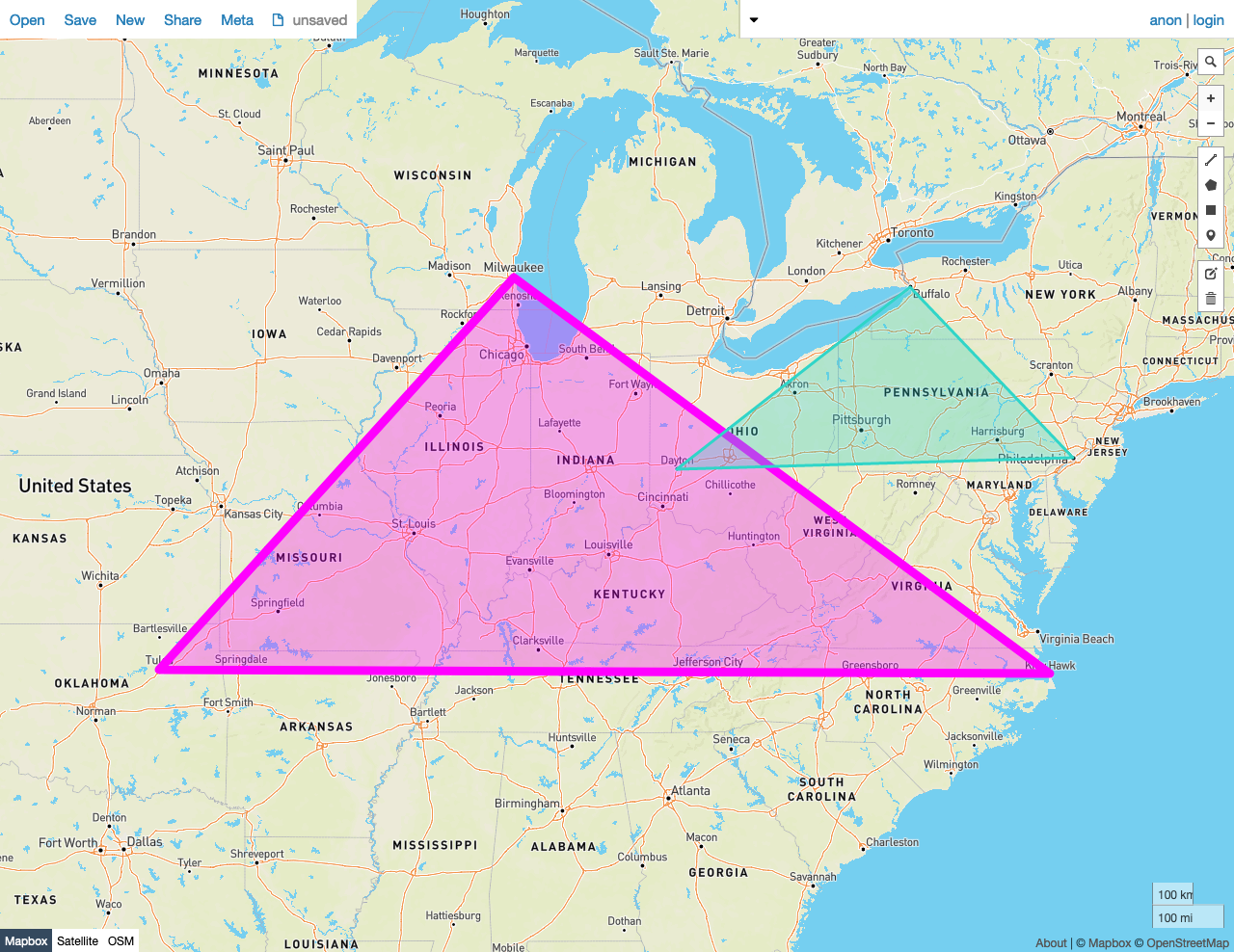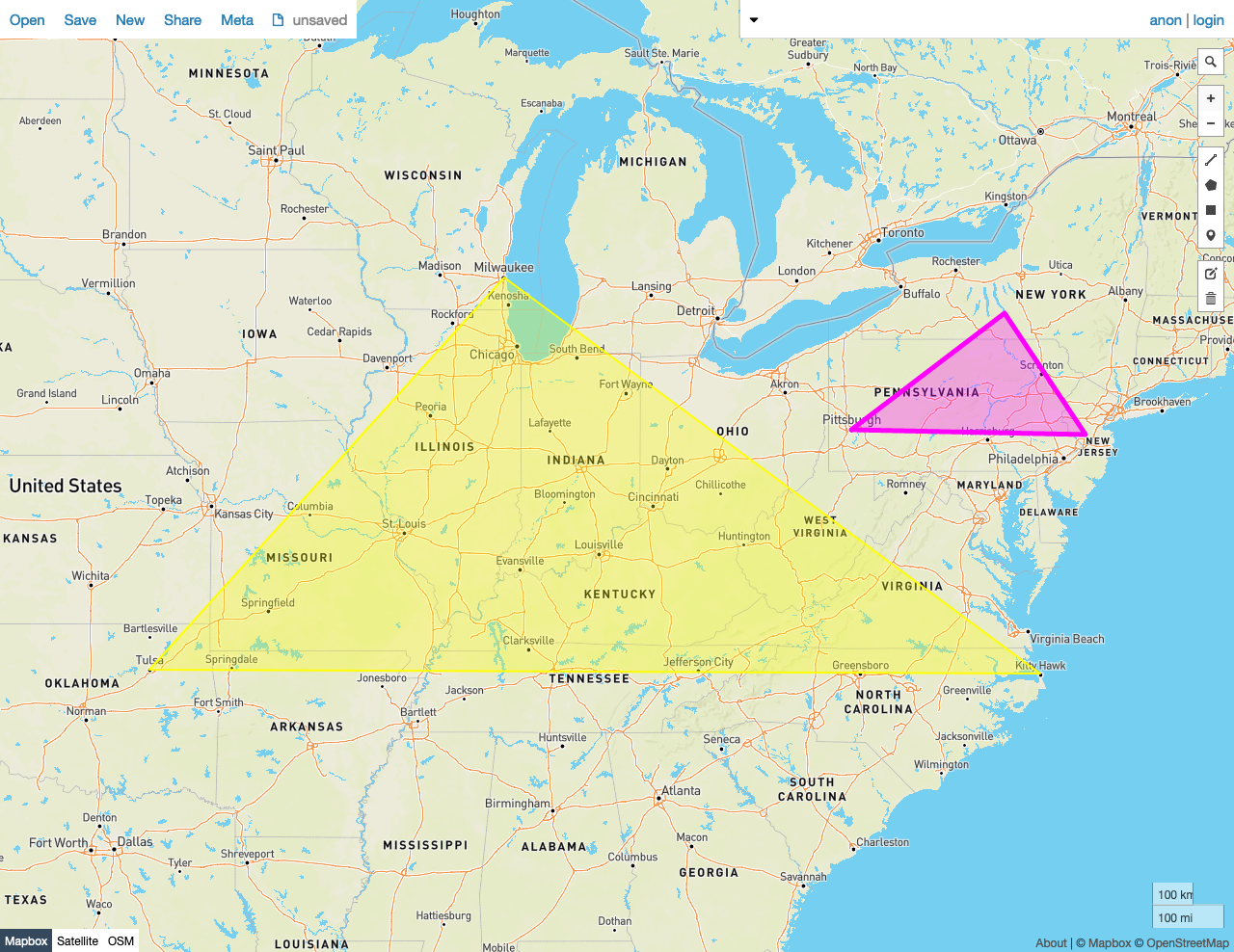On this pageWarning:
Cockroach Labs will stop providing Assistance Support for v22.1 on November 24, 2023. Prior to that date, upgrade to a more recent version to continue receiving support. For more details, see the Release Support Policy.

Given two shapes A and B, `ST_Intersects(A, B)` returns `true` if the shapes share any of the same space -- that is, if any point in the set that comprises A is also a member of the set of points that make up B.

`ST_Intersects` works on the following data types:

Note:

`ST_Intersects` will attempt to use any available spatial index to speed up its operation. Use the prefixed variant `_ST_Intersects` if you do not want any spatial indexes to be used.

Note:

This function is the inverse of `ST_Disjoint`.

## Examples

Note:

The screenshots in these examples were generated using geojson.io, but they are designed to showcase the shapes, not the map. Representing `GEOMETRY` data in GeoJSON can lead to unexpected results if using geometries with SRIDs other than 4326 (as shown below).

### True

In this example, `ST_Intersects` returns `true` because:

• The shapes share some of the same space -- that is, there are Points in the set that comprises Polygon A that are also members of Polygon B.
``````SELECT st_intersects(st_geomfromtext('SRID=4326;POLYGON((-87.906471 43.038902, -95.992775 36.153980, -75.704722 36.076944, -87.906471 43.038902))'), st_geomfromtext('SRID=4326;POLYGON((-84.191605 39.758949, -75.165222 39.952583, -78.878738 42.880230, -84.191605 39.758949))'));
``````
`````` st_intersects
---------------
true

(1 row)
``````### False

In this example, `ST_Intersects` returns `false` because:

• The shapes do not share any of the same space -- that is, there are no Points in the set that comprises Polygon A that are also members of Polygon B.
``````SELECT st_intersects(st_geomfromtext('SRID=4326;POLYGON((-87.906471 43.038902, -95.992775 36.153980, -75.704722 36.076944, -87.906471 43.038902))'), st_geomfromtext('SRID=4326;POLYGON((-79.995888 40.440624,-74.666728 40.358244, -76.5 42.443333, -79.995888 40.440624))'));
``````
`````` st_intersects
---------------
false
(1 row)
``````